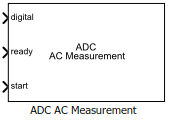Measure AC performance metrics of ADC output

• Library:
• Mixed-Signal Blockset / ADC / Measurements & Testbenches

•## Description

The ADC AC Measurement block measures ADC AC performance metrics such as signal to noise ratio (SNR), signal to noise and distortion radio (SINAD), spurious free dynamic range (SFDR), effective number of bits (ENOB), noise floor, and conversion delay. You can use ADC AC Measurement block to validate the ADC architectural models provided in Mixed-Signal Blockset™, or you can use an ADC of your own implementation

## Ports

### Input

expand all

Converted digital signal from an ADC, specified as a scalar.

Data Types: `fixed point` | `single` | `double` | `int8` | `int16` | `int32` | `uint8` | `uint16` | `uint32`

Indicates whether the analog to digital conversion is complete, specified as a scalar.

Data Types: `double`

External conversion start clock, specified as a scalar. The analog to digital conversion process starts at the rising edge of the signal at the start port.

Data Types: `double`

## Parameters

expand all

Type of distortion the ADC AC Measurement block is set to measure, specified as `Harmonic` or `Intermodulation`.

#### Programmatic Use

 Block parameter: `DistortionMeasurement` Type: character vector Values: `Harmonic` | `Intermodulation` Default: `Harmonic`

Frequency of the analog input signal to an ADC block, specified as a positive real scalar in hertz. Analog stimulus frequency must match the input frequency to the ADC device under test.

• When the ADC AC Measurement is set to measure the `Harmonic` distortion, the default value of Analog stimulus frequency is `10000`.

• When the ADC AC Measurement is set to measure the `Intermodulation` distortion, the default value of Analog stimulus frequency is `[9000,11000]`.

Analog stimulus frequency needs to satisfy two requirements:

• All the output codes of the ADC must be activated.

• The Analog stimulus frequency must not share any common multiples other than 1 with the Start conversion frequency.

To satisfy both the conditions, use the equation ${f}_{\text{analog}}=\frac{J}{M}{f}_{\text{start}}$ ,

where:

fanalog is the analog signal frequency,

fstart is the start conversion frequency,

$M>{2}^{Nbits}·\pi$, where Nbits is the number of bits of the ADC,

and J is an integer with no common factors with M.

#### Programmatic Use

 Block parameter: `InputFrequency` Type: character vector Values: positive real scalar | positive real valued vector Default: `10000`

Resolution bandwidth, specified as a positive real scalar in hertz. This parameter defines the smallest positive frequency that can be resolved. By default, this parameter is calculated automatically. You can deselect Set automatically to customize the value.

• When the ADC AC Measurement is set to measure the `Harmonic` distortion, the default value of Resolution bandwidth (Hz) is `1000`.

• When the ADC AC Measurement is set to measure the `Intermodulation` distortion, the default value of Resolution bandwidth (Hz) is `900`.

#### Programmatic Use

 Block parameter: `RBW` Type: character vector Values: positive real scalar Default: `1000`

Number of physical bits in ADC, specified as a unitless positive real integer. Number of bits must match the resolution specified in the ADC block.

#### Programmatic Use

 Block parameter: `NBits` Type: character vector Values: positive real integer Default: `5`

Frequency of the start conversion clock of the ADC, specified as a positive real scalar in hertz. Start conversion frequency must match the frequency of the start conversion clock of the ADC block.

#### Programmatic Use

 Block parameter: `Frequency` Type: character vector Values: positive real scalar Default: `10e6`

Delays measurement analysis to avoid corruption by transients, specified as a nonnegative real scalar in seconds.

#### Programmatic Use

 Block parameter: `HoldOffTime` Type: character vector Values: nonnegative real scalar Default: `0`

Minimum time the simulation must run to obtain meaningful results, specified as a positive real scalar in seconds.

For AC measurement, the simulation must run so that the ADC can generate 6 spectral updates of the ADC output. The time to generate one spectral output based on Welch's method  is given by:

`$t=\frac{1.5·SamplingFrequency}{RBW}$`

where SamplingFrequency and RBW are the sampling frequency and resolution bandwidth of the spectrum estimator inside the ADC Testbench block.

This parameter is only reported by the testbench and is not editable.

Data Types: `double`

Click to automatically set the Recommended min. simulation stop time (s) as the stop time of the Simulink® model.

Store detailed test results in the base workspace for further processing at the end of simulation. By default, this option is not selected.

Name of the variable that stores detailed test results, specified as a character string.

#### Dependencies

This parameter is only available when Output result to base workspace is selected.

#### Programmatic Use

 Block parameter: `VariableName` Type: character vector Values: character string Default: `adc_ac_out`

Displays spectrum analyzer during simulation. By default, this option is not selected.